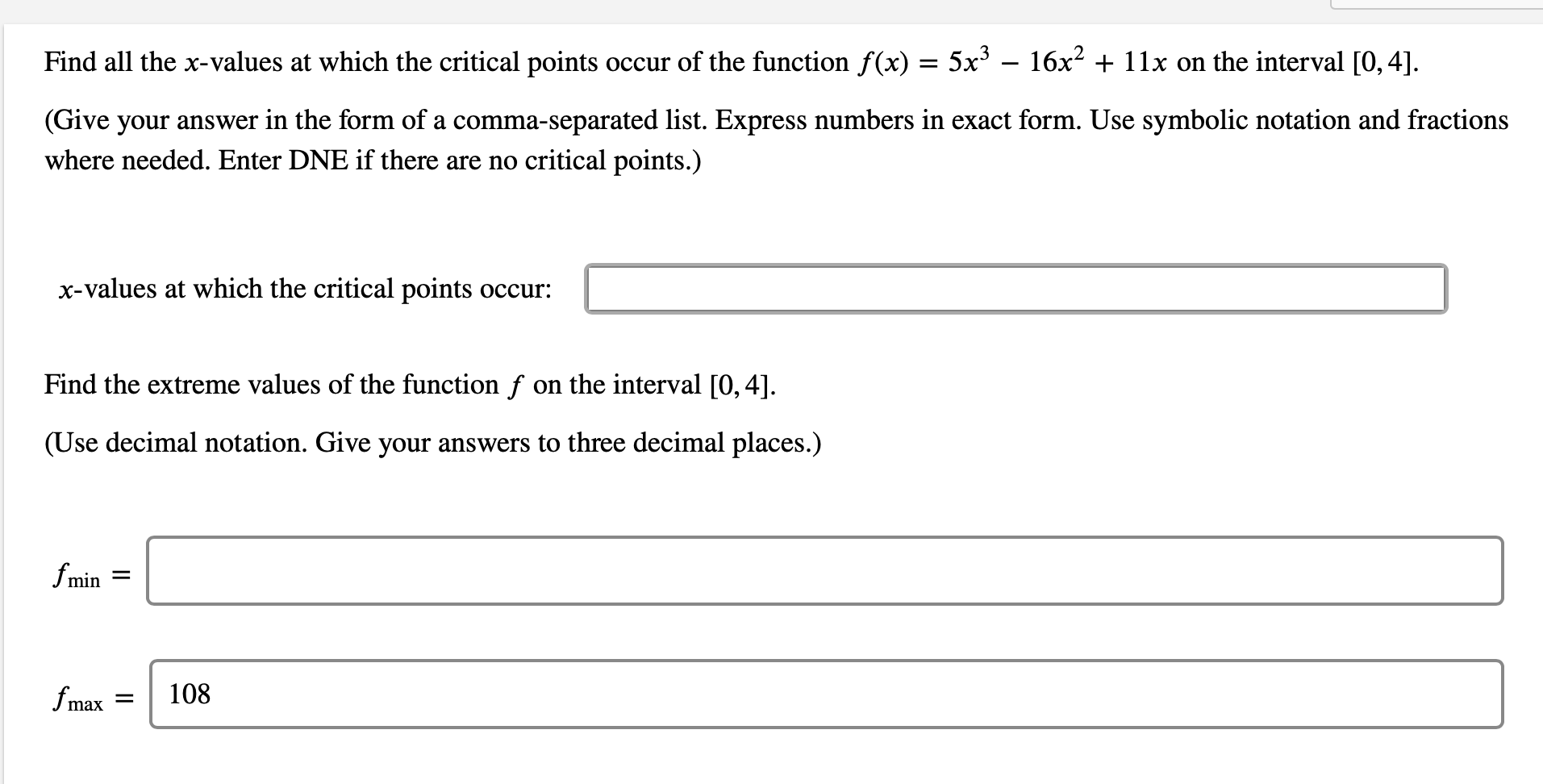# Find all the x-values at which the critical points occur of the function f(x) = 5x – 16x2 + 11x on the interval [0, 4]. (Give your answer in the form of a comma-separated list. Express numbers in exact form. Use symbolic notation and fractions where needed. Enter DNE if there are no critical points.) x-values at which the critical points occur: Find the extreme values of the function f on the interval [0, 4]. (Use decimal notation. Give your answers to three decimal places.) fmin = fmax 108

Questionhelp_outlineImage TranscriptioncloseFind all the x-values at which the critical points occur of the function f(x) = 5x – 16x2 + 11x on the interval [0, 4]. (Give your answer in the form of a comma-separated list. Express numbers in exact form. Use symbolic notation and fractions where needed. Enter DNE if there are no critical points.) x-values at which the critical points occur: Find the extreme values of the function f on the interval [0, 4]. (Use decimal notation. Give your answers to three decimal places.) fmin = fmax 108 fullscreen

### Want to see this answer and more?

Experts are waiting 24/7 to provide step-by-step solutions in as fast as 30 minutes!*

*Response times vary by subject and question complexity. Median response time is 34 minutes and may be longer for new subjects.
Tagged in
MathCalculus

### Applications of Derivative